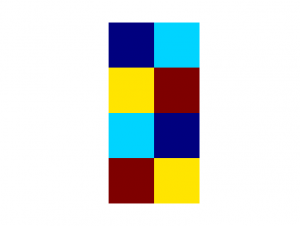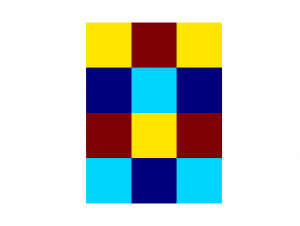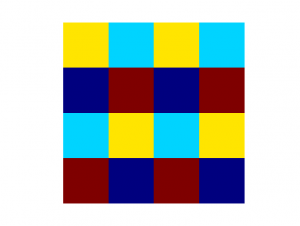# Tiling Problem

We have a play-mat: eight rubber tiles, two of each colour, that fit together. We had put it together in a 4x2 arrangement, without considering the colours. The result was ... displeasing. Two pink tiles were right next to each other, but all the other pairs were separated. It just looked wrong. Eventually I had to adjust it. Here's the "most correct" looking arrangement I came up with:It appears to be the arrangement in which tiles of the same colour are as fair apart from each other as possible. I wondered if this "rule" could be used to automatically generate the most aesthetically pleasing arrangement.

The first issue was how to measure how "far apart" tiles of the same colour are. For each same-coloured pair, I calculated a distance, based on their grid positions. (Adjacent tiles have a distance of 1, diagonal tiles have a distance of sqrt(2).) But how should we combine the measurements for all the pairs.

At first I tried taking the minimum separation for each colour, and then averaging across all the colours. This didn't have the desired effect, though, as one colour with a very large separation would outweigh the other colours being closer together. And, aesthetically, a more symmetric outcome seemed correct.

Switching instead to minimizing the distances across all colours led to the symmetric solution (above) for the 4x2 arrangement. Great! Let's try it with a larger grid now, and see what we get.

But now we run into the second issue. I've been finding the optimum solution by listing all the permutations of the tiles, calculating the distance measure for each permutation, and then returning (one of) the permutations with the minimum measure. This works fine for 4x2, 5x2, but for anything beyond this, it's too slow.

First, I tired implementing a more efficient partition algorithm (rather than listing all the permutations, as many of the permutations correspond to identical tile-colour patterns). This was faster; however, since the partition algorithm was recursive, I quickly hit the Python stack limit for larger grids.

Rather than trying to refactor the partition algorithm, this seemed like a sign that I should stop trying to brute-force all the different options, and switch to an optimization algorithm.

Simulated Annealing seemed like a good option, for a discrete optimization problem like this one. However, for larger grids, the results would be curiously diagonal or vertical stripes (depending on the exact situation). These turned out to be the arbitrary starting arrangement that I was feeding into the algorithm. Nothing was changing at all.

After a lot of checking of my implementation, the culprit turned out to be the distance measure that I had chosen for the previous algorithm. Because I was taking minimums, and the initial configuration was symmetric, no single change to the arrangement resulted in any change to the distance measure. Many tiles were the same distance apart, and while moving two of them farther away would bring us "closer" to the correct solution, this was not reflected in the distance measure, since the minimum distance between two tiles of the same colour remained unchanged.

Clearly we need a better distance measure. Just a regular average favoured a single large distance and didn't penalize many other smaller distances enough. Taking the minimum penalized the smaller distances, but isn't sensitive enough to detect small improvements. An inverse square measure seemed like an appropriate compromise between these two (although the problem had to be changed to now minimize this measure, rather than maximizing the distance in the previous two cases).

With the new improved inverse square simulated annealing set-up, we can now expand our four colours onto a 4x3 grid:And a 4x4 grid:Both results keep the original 4x2 pattern, and just add new columns onto it. It's interesting that the 4x4 arrangement isn't even close to rotationally symmetric.

To come: more grid configurations, and different numbers of colours. And then we can see about tackling this symmetry thing.